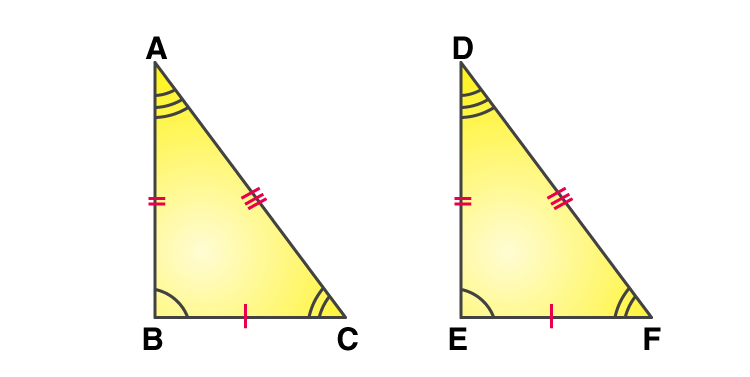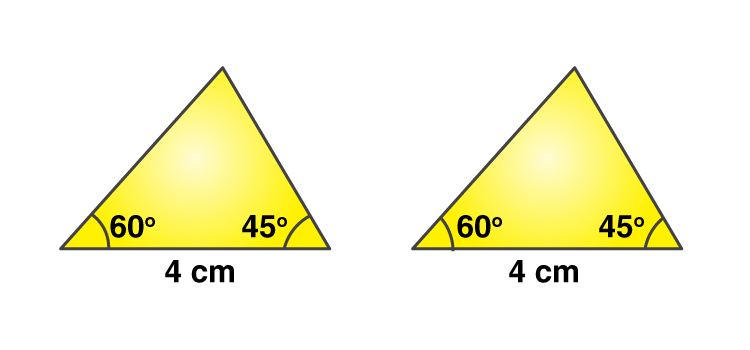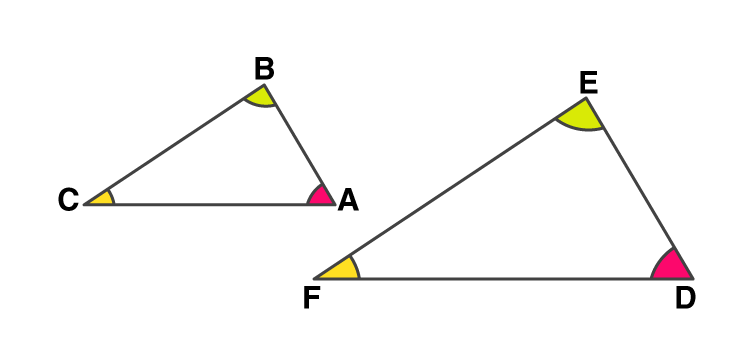# Triangles Class 9 Notes - Chapter 7

## CBSE Class 9 Maths Triangles Notes:-

Triangles for class 9 notes are given here. The complete notes for the concept of triangles cover the following concepts such as congruency, criteria for the congruency such as SAS, SSS, ASA, AAS, RHS are explained here in detail.

### Congruent Triangles

In a pair of triangles if all three corresponding sides and three corresponding angles are exactly equal, then the triangles are said to be congruent.In congruent triangles, the corresponding parts are equal and are written as CPCT (Corresponding part of the congruent triangle).

To know more about Congruency of Triangles, visit here.

## Criteria for Congruency

The following are the criteria for the congruency of the triangles.

### SSS Criteria for Congruency

• If three sides of one triangle are equal to the three sides of another triangle, then the two triangles are congruent.
• If all sides are exactly the same, then their corresponding angles must also be exactly the same.

### SAS Criteria for Congruency

– Axiom: Two triangles are congruent if two sides and the included angle of one triangle are equal to the corresponding sides and the included angle of the other triangle.

### ASA Criteria for Congruency

– Two triangles are congruent if two angles and the included side of one triangle are equal to the corresponding two angles and the included side of the other triangleAn included side is 4cm

### AAS Criteria for Congruency

– Two triangles are said to be congruent to each other if two angles and one side of one triangle are equal to two angles and one side of the other triangle.

### Why SSA and AAA congruency rules are not valid?

• SSA or ASS test is not a valid test for congruency as the angle is not included between the pairs of equal sides.-
• The AAA test also is not a valid test as even though 2 triangles can have all three same angles, the sides can be of differing lengths. This becomes a test for similarity (AA).Angles of a triangle

### RHS Criteria for Congruency

• If in two right triangles the hypotenuse and one side of one triangle are equal to the hypotenuse and one side of the other triangle, then the two triangles are congruent.
• RHS stands for Right angle – Hypotenuse – Side.

### Properties of Isosceles triangle

– If 2 sides of the triangle are equal, the angles opposite those sides are also equal and vice versa.

To know more about Properties of Isosceles Triangle, visit here.

### Criteria for Congruency of triangles

– The criteria for congruency of triangles are :

• SAS
• ASA
• AAS
• SSS
• RHS

symbolically, it is expressed as ΔABCΔXYZ

## Inequalities in Triangles

### Relationship between unequal sides of the triangle and the angles opposite to it.

– If 2 sides of a triangle are unequal, then the angle opposite to the longer side will be larger than the angle opposite to the shorter side.

### Triangle inequality

– The sum of the lengths of any two sides of a triangle must be greater than the third side.

To know more about Triangles Inequalities Theorem, visit here.

Quiz on Triangles Class 9

2. Mehver Bashir

It’s so easy and useful

3. BEST WEBSITE FOR LEARNING.

4. Shivanshu Singh

Very Easy to Revise during tests and exams

5. Aaryan Rai

Thanks for such an understandable info

6. Tiger Shroff

Very nice website and a trusted website easy to learn from here .

7. ilankathir

thanks for the notes

8. Ayushi arya

It’s very helpful ..for me thanx byjus

9. it so use full

10. Thank you very much. It is very useful

11. Kanishka

It’s nice platform to read easy and easy notes

12. Its really help ful and very easy to understand

13. That was amazing !! Learning app

14. very useful for writing notes